Select Page

# Special Application of Time Value of Money Problems and Solutions

## Problem 1:

A firm wants to open a new coal mine. The price of coal is very volatile and the projected profits over the next five years are : Rs. 100,000  ,  Rs. 250,000  ,  Rs. 10,000  ,  Rs. 200,000  and  Rs. 50,000 respectively. After that profits will be a constant Rs. 150,000 per year for next 20 years at which time the mine closes. If 7% is the appropriate discount rate for the first five years and is 8% after that, what is the present value of the mine?

### Solution: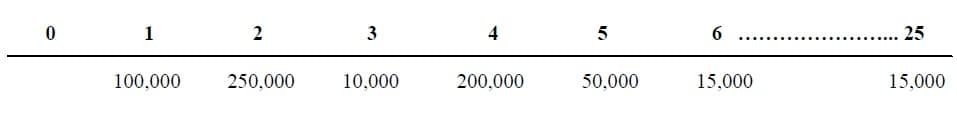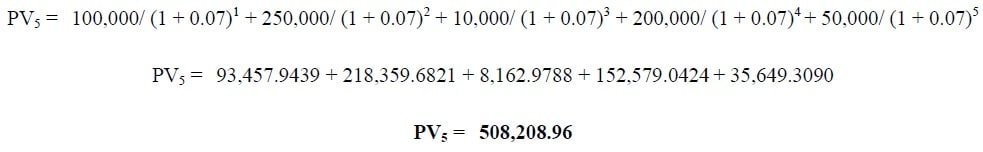##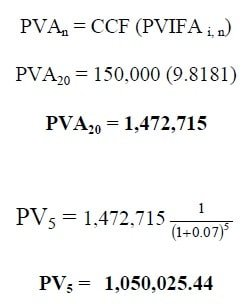Total Present Value = 508,208.96 + 1,050,025.44

# Special Application of Time Value of Money Problems and Solutions

## Problem 1:

A firm wants to open a new coal mine. The price of coal is very volatile and the projected profits over the next five years are : Rs. 100,000  ,  Rs. 250,000  ,  Rs. 10,000  ,  Rs. 200,000  and  Rs. 50,000 respectively. After that profits will be a constant Rs. 150,000 per year for next 20 years at which time the mine closes. If 7% is the appropriate discount rate for the first five years and is 8% after that, what is the present value of the mine?

### Solution:##Total Present Value = 508,208.96 + 1,050,025.44

## Problem 2:

You are borrowing Rs. 80,000 for 25 years at 10% nominal annual interest.  How much must your annually payments be if you will completely retire the loan over the 25-year period by factor formula?

## Problem 3:

Perpetuity makes payments of Rs. 500 every year, with the first payment coming one year from today. If the discount rate is 5%, what is the present value of the perpetuity?

## Problem 4:

Waleed just purchased a new house for Rs. 120,000. He was able to make a down payment equal to 25% of the value of the house; the balance was mortgaged. The rate by the bank is 10% compounded annually. The mortgage has a 20 year amortization period (this means that payments are calculated assuming it will take 20 years to pay off the loan).

(a) What will be the size of the payments by factor formula?

(b) What will be the balance remaining on the mortgage after 5 years?

### Solution:

Down Payment = 120,000* 25%   = Rs. 30,000

Loan Amount = 120,000 – 30,000   = Rs. 90,000

##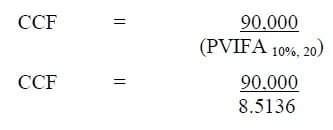CCF = Rs. 10,571.32

##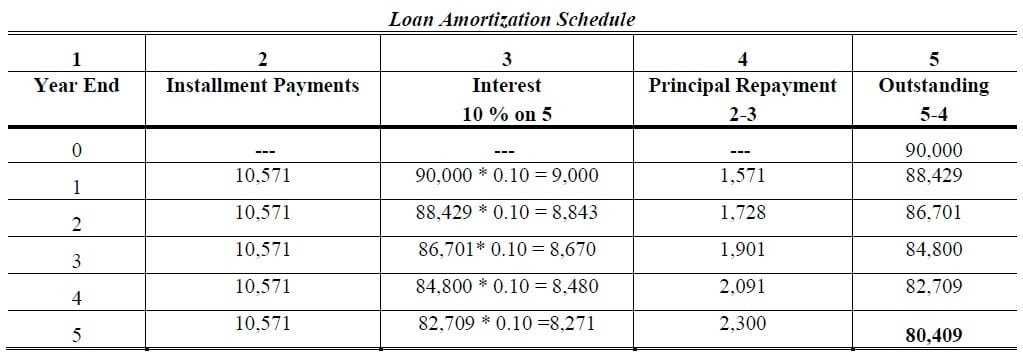Answer: (a) Rs. 10,571.32      (b) Rs. 80,409

## Problem 5:

If you borrow Rs. 150,000 for a house at 8% compound annual interest rate for 30 years, what is your monthly payment by general formula?

### Solution: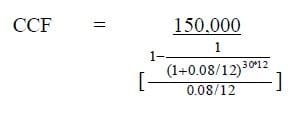## Problem 6:

An annual interest rate of 12% compounded monthly has an effective yield of?

## Problem 7:

An annual interest rate of 12% compounded quarterly has an effective yield of?

## Problem 8:

A simple annual interest rate of 12% compounded semi-annually is an effective yield of?

## Problem 9:

A real estate investor feels that the cash flow from a property will enable his to pay a lender Rs. 15,000 per year, at the end of every year, for 10 years.  How much should the lender be willing to loan her if he requires a 9% annual interest rate by general formula (annually discounting, assuming the first of the 10 equal payments arrives one year from the date the loan is disbursed)?

## Problem 10:

Now that you are finished school, you also have to start paying back your student loans. You borrowed a total of Rs. 12,500. You plan to pay back the loan over 10 years at an interest rate of 9.4% interest, compounded monthly. How much will your monthly payments be?

## Related Topics

Formula Sheet

Future Value and Present Value Tables

## Related Courses

Principles of Accounting

Cost Accounting

Principles of Finance